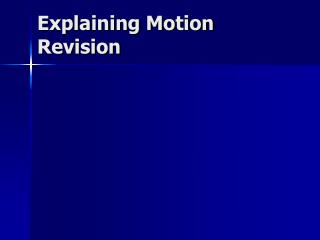DownloadDownload PresentationExplaining Motion Revision

# Explaining Motion Revision

Télécharger la présentation## Explaining Motion Revision

- - - - - - - - - - - - - - - - - - - - - - - - - - - E N D - - - - - - - - - - - - - - - - - - - - - - - - - - -
##### Presentation Transcript

1. Explaining Motion Revision

2. Forces • Forces arise from an interaction between 2 objects. • In an interaction pair the forces are equal in size but opposite in direction. • These forces act on different objects. • They explain how rockets work etc.

3. Forces (cont.) • Draw diagrams showing forces with arrows. • Some forces are a response to other forces eg friction, reaction.

4. Forces (cont.) • The resultant force on an object is the sum of all the individual forces acting on it • All forces are in pairs

5. Speed • Average speed = distance/time • Distinguish between average speed and instantaneous speed. • Remember that movement in one direction is positive and the other direction is negative.

6. Distance-Time Graphs • Draw and interpret graphs that show objects that are • Stationary • Moving at constant speed • Increasing or decreasing speed • Recall that a steeper gradient means a faster speed. • Make calculations from a distance-speed graph.

7. Velocity-Time Graphs • Recall that velocity is speed with an indication of direction. • Interpret velocity-time graphs for objects that are • Stationary. • Moving in a straight line at steady speed. • Moving in a straight line with changing speed. • Be able to read a tachograph.

8. Momentum • Momentum = mass x velocity • When a force acts on an object it causes a change in momentum in the direction of the force. • The size of the change in momentum is related to the size of the force and the time it was applied. • Change in momentum= force x time

9. Changing momentum safely • In a collision, if the time taken is increased that size of the force will be smaller – causing less damage. • This is how air bags, seat belts, crumple zones etc work

10. Forces and Motion • Motion can be described in terms of a driving force and a counter force. • The driving force is the engine or pedals. • The counter force is air resistance and the brakes. • If the driving force is • Greater than the counter force the object speeds up • Equal to the counter force the object moves at constant speed (or remains stationary). • Less than the counter force the object slows down.

11. Energy Changes • Moving objects have kinetic energy • KE= ½ mv2. • Objects that are lifted have gravitational potential energy. • GPE=weight x change in height. • When on object does work it loses energy. • When work is done on an object it gains energy. • Change in energy = work done.

12. Energy Changes • When a force causes an object to move, work is done. • Work done = force x distance. • Lifting an object gives it GPE, moving an object gives it KE. • The bigger or faster an object the greater the KE. • The greater or higher an object the greater the GPE

13. Energy Changes • A falling object loses GPE but gains KE. • The rise in KE is equal to the fall in GPE (assuming no air resistance or friction). • Air resistance and friction will stop the gain in KE being equal to the work done.